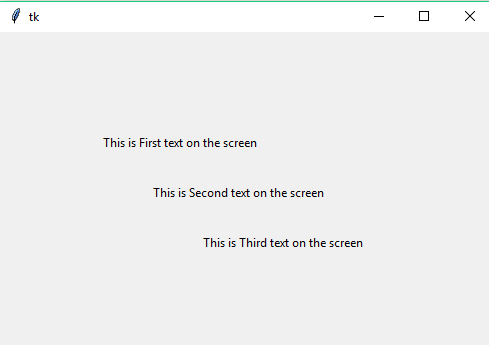# Label widget in tkinter by R4R Team

program-

from tkinter import *

win=Tk()

l=Label(win,text="This is First text on the screen")
l.place(x=100,y=100)

l=Label(win,text="This is Second text on the screen")
l.place(x=150,y=150)

l=Label(win,text="This is Third text on the screen")
l.place(x=200,y=200)

win.mainloop()

output--In this program, firstly we create a window screen by the win=Tk() where Tk() is the constructor and win is the object variable choosen by the programmer.
- we create a label text by Label widget in the tkinter by Label(win,text="string")

-We place the Label or text at the screen by the l.place(x,y)
-where x and y are the coordinate